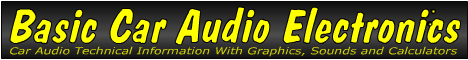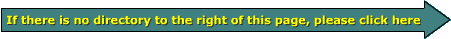Voltage

As we said on the previous page, if work is to be done, something has to move. For something to move, a force has to be applied to the object. In electronics, that force is voltage. Voltage is the measure of difference of potential (electrical force) between two points. The volt is the unit of measure. Voltage can be described as electrical pressure.

Water Analogy:
To relate this to something that you already understand well, the water analogy will be used here. If you've ever gone swimming in a deep pool and went to the bottom where it was approximately 8 feet deep, you felt the increased pressure on your body and especially on your ears. This is due to the weight of the column of water that's above you. This is the same principal that municipal water companies use to produce and maintain water pressure in the pipes that feed your home. For water towers, the pressure is directly proportional to the height of the column of water in the tank.

Pressure in the tank and the column of water depends on where you are in the column. At the top of the column of water, there is no pressure because there is no water above that point. At the middle of the water column, the pressure is half of the pressure at the bottom of the column. If you think about it a bit more, you'll realize that when you measure anything, you need a reference. For water pressure, you would use the top of the column (0 pressure) as the reference. Let's say that the water column is about 100 feet tall. That would mean that the pressure at the bottom of the column is about 40psi (pounds of pressure per square inch). This would mean that the pressure in the middle of the water column is about 20psi.

Below, you can see that there are two batteries next to the water tower. The batteries produce a sort of pressure, electrical pressure or 'voltage'. Here each battery is 6 volts. Again, when you measure anything, you need a reference. Conveniently here, 'ground' is the reference. At the top of the first battery, you have 6 volts (referenced to the 0v/ground). At the top of the top battery, the voltage is 12 volts.

• You should remember:
• Voltage is electrical force.

```

```